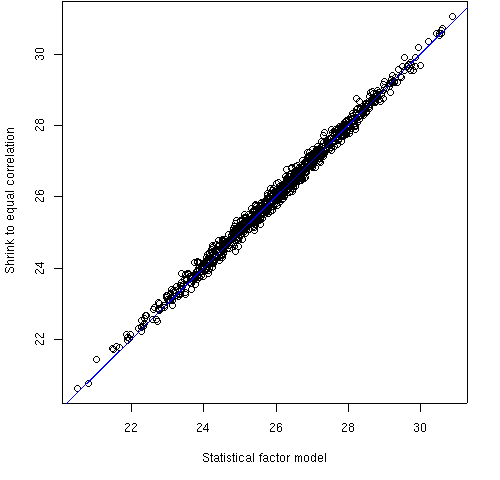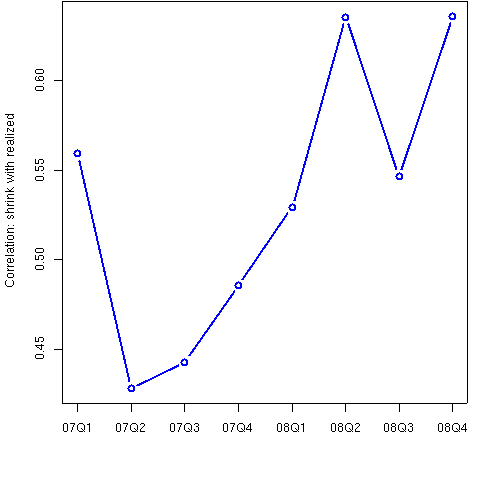## Assess Risk Models

### Summary

Create realistic portfolios with which to test and compare risk models.

### Description

You can generate random portfolios that have constraints similar to the portfolios that you actually hold. With such portfolios you can:

• Compare risk models (see Figure 1).
• Test model predictions versus realized values (see Figure 2).
• Look for weak spots in models by varying the constraints.

Figure 1: Predicted volatility from two risk modelsFigure 1 compares the predictions from two risk models of 1000 equity portfolios with a certain set of constraints. One of the models is a statistical factor model, the other shrinks the sample variance towards the equal correlation matrix.

Figure 2: Correlation of predicted versus realized volatilityFigure 2 shows the correlation between the predicted volatility of a risk model (the shrinkage model) and the realized volatility for eight quarters. There are 1000 portfolios in each quarter. The portfolios are long-short dollar neutral.